## 机器学习笔记02——线性回归

news/2023/9/22 15:15:17
• 周志华《机器学习》第三章
• https://blog.csdn.net/weixin_45666566/article/details/107137877      其他up主博客总结

1.1 步骤分析

1.2 代码过程

3.1 正规方程进行波士顿房价预测

3.2 梯度下降法进行波士顿房价预测

​​​​​梯度下降法API：

七、维灾难

8.1 岭回归（Ridge Regression）

8.2 LASSO回归

8.3 Elastic Net弹性网络

8.4 Early stopping [了解]

8.5 岭回归的应用——波士顿房价预测

波士顿房价预测——Ridge、RidgeCV：

## 一. 线性回归API初步使用

• sklearn.linear_model.LinearRegression()
• LinearRegression.coef_ : 返回回归系数

sklearn.linear_model.LinearRegression — scikit-learn 1.1.3 documentationhttps://scikit-learn.org/stable/modules/generated/sklearn.linear_model.LinearRegression.html#sklearn.linear_model.LinearRegression

• 1.获取数据
• 2.数据基本处理
• 3.特征工程（标准化）
• 4.机器学习（线性回归）
• 5.模型评估（均方误差）

### 1.2 代码过程

• 导入模块
from sklearn.linear_model import LinearRegression

• 构造数据集
# 获取数据
x= [
[80,86],
[82,80],
[85,78],
[90,90],
[86,82],
[82,90]
]
y=[84.2,80.6,80.1,90,83.2,87.6]

• 模型训练
• 实例化一个估计器
• 调用fit方法
# 1.实例化一个估计器
estimator = LinearRegression()
# 2.调用fit方法
estimator.fit(x,y)
# 3.查看一下系数值
coef = estimator.coef_
print('系数是：\n',coef)


## 二、优化算法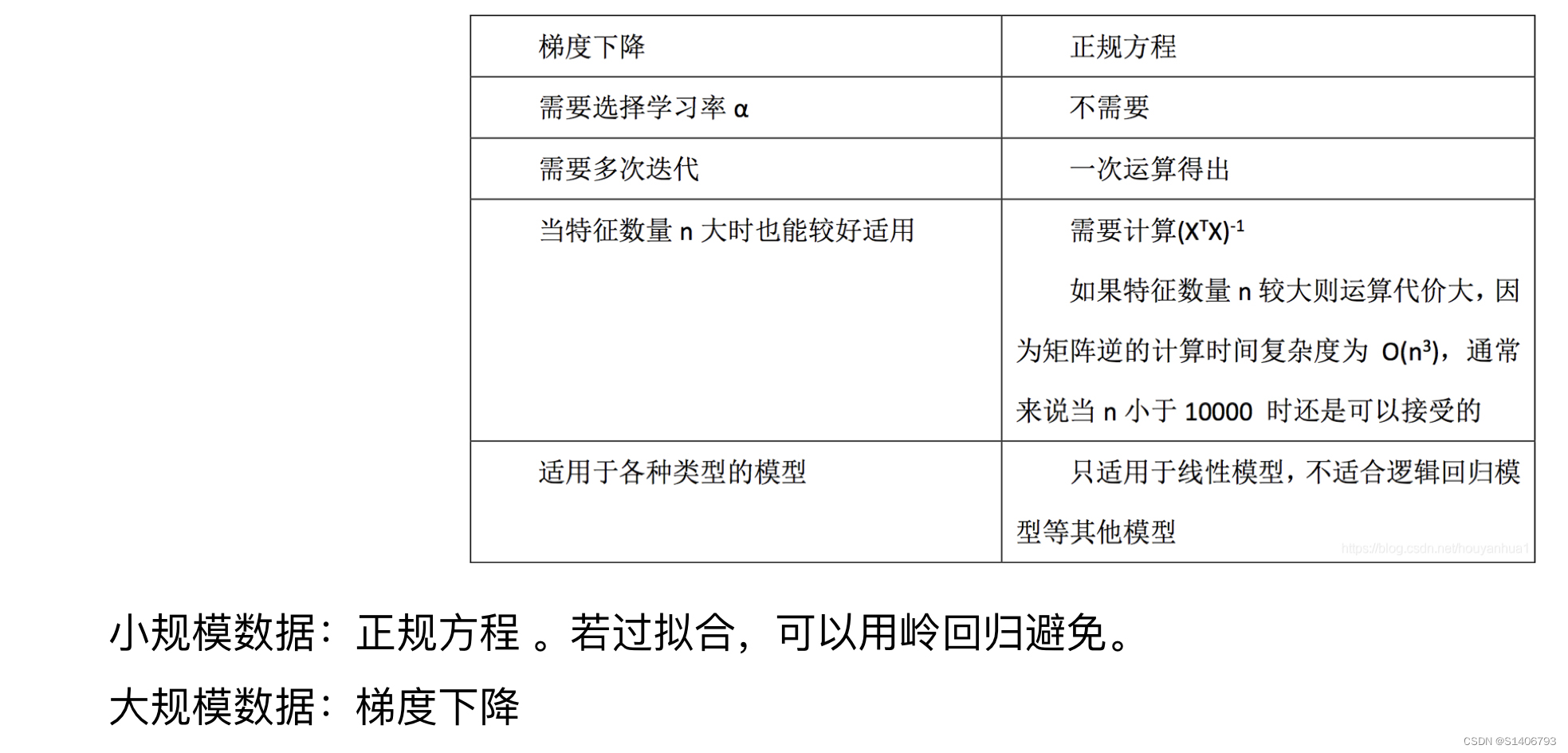# 三、案例：波士顿房价预测

1. 回归当中数据大小不一致，是否会导致结果影响较大，所以要做标准化处理

• 数据分割与标准化处理
• 回归预测
• 线性回归的算法效果评估

2. 回归性能评估

sklearn.metrics.mean_squard_error(y_true,y_pred)

## 3.1 正规方程进行波士顿房价预测

正规方程API:

• sklearn.linear_model.LinearRegression(fit_intercept = True)
• 正规方程优化
• fit_intercept：是否计算偏置
• LinearRegression.coef_ ：回归系数
• LinearRegression.intercept_：求偏置是什么
# 正规方程
import pandas as pd
import numpy as np
from sklearn.model_selection import train_test_split  #数据集划分
from sklearn.preprocessing import StandardScaler  #特征工程标准化
from sklearn.linear_model import LinearRegression  #机器学习
from sklearn.metrics import mean_squared_error    #模型评估

def linear_model1():
'''
正规方程
'''
# 1.获取数据

# 2.数据基本处理
# 2.1 数据集划分
x_train,x_test,y_train,y_test = train_test_split(boston.data,boston.target,test_size=0.2)

# 3.特征工程 --标准化(实例化，fit_transform)
transfer  = StandardScaler()
x_train = transfer.fit_transform(x_train)
x_test = transfer.fit_transform(x_test)

# 4.机器学习（线性回归）
estimator = LinearRegression()
estimator.fit(x_train,y_train)
print('模型的偏置是:\n',estimator.intercept_)

# 5.模型评估
# 5.1 预测值和准确率
y_pre = estimator.predict(x_test)
print('预测值似乎:\n',y_pre)
score = estimator.score(x_test,y_test)
print('准确率是:\n',score)

# 5.2 均方误差评估
ret = mean_squared_error(y_test,y_pre)
print('均方误差是:\n',ret)

if __name__='__main__':
linear_model1()
模型的偏置是:
22.590099009901042

[16.18423286  9.4826414  29.97223094 20.92230567 20.18119974 28.0550033
39.09520032 34.67667493  9.13493858 10.79386927 24.29023285 25.82819773
20.90636029 12.24051743 11.97957095 20.77288158 15.86035645 24.28428371
...]

0.7619097471422422

22.87140829473221


## 3.2 梯度下降法进行波士顿房价预测

### ​​​​​梯度下降法API：

• sklearn.linear_model.SGDRegression(loss=‘squard_loss’,fit_intercept=True,learning_rate=‘invscaling’,eta0=0.01)
• SGDRegression 实现了随机梯度下降学习，支持不同的Lasso函数和正则化惩罚项来拟合线性回归模型
• loss：损失类型
• loss=‘squard_loss’：普通最小二乘法
• fit_intercept:是否计算偏置
• learning_rate: string，optional
• 学习率填充
• ‘constant’：eta=eta0 — 常数，后期效果不好
• ‘optimal’：eta=1.0/(alpha*(t+t0))[default] — 默认，随着t的增大eta变小
• ‘invscaling’：eta=eta0/pow(t,power_t)
-POWER_T=0.25:存在父类当中
• 对于一个常数值的学习率来说，可以使用learning_rate = ‘constant’，并使用eta0来指定学习率。
• SGDRegressor.coef_：回归系数
• SGDRegressor.intercept_：偏置2.数据基本处理
• -2.1 数据集划分
• 3.特征工程 --标准化
• 4.机器学习（线性回归）
• 5.模型评估
import pandas as pd
import numpy as np
from sklearn.model_selection import train_test_split  #数据基本处理
from sklearn.preprocessing import StandardScaler  #特征工程
from sklearn.linear_model import LinearRegression, SGDRegressor    #机器学习（梯度下降法）
from sklearn.metrics import mean_squared_error   #模型评估

def linear_model2():
'''
梯度下降法
'''
# 1.获取数据

# 2.数据基本处理
# 2.1数据集划分
x_train,x_test,y_train,y_test = train_test_split(boston.data, boston.target, test_size = 0.2)

# 3.特征工程: 实例化＋fit_transform方法
transfer = StandardScaler(x_train,x_test)
x_train = transfer.fit_transform(x_train)
x_test = transfer.fit_transform(x_test)

# 4.机器学习（线性回归）
estimator = SGDRegressor(max_iter=1000,learning_rate = 'optimal')  #最大迭代次数1000
estimator.fit(x_train,y_train)
print('这个模型的偏置是：\n',estimator.intercept_)

# 5.模型评估
# 5.1 预测值和准确率
y_pre = estimator.predict(x_test)
print('预测值是：\n',y_pre)

score = estimator.score(x_test,y_test)
print('准确率是:\n',score)

# 5.2均方误差
ret = mean_squared_error(y_test,y_pre)
print('均方误差是：\n',ret)

if __name__ == '__main__':
linear_model2( )

• 我们可以尝试着去修改学习率

estimator = SGDRegressor ( max_iter=1000, learning_rate=‘constant’, eta0=0.1 )

## 四、 非线性回归

• 例如二次函数、三次函数等等
• 以后会讨论一些算法，能够自动选择要使用什么特征，观察数据并自动为我们选择什么函数。

# 五、欠拟合和过拟合

• 欠拟合原因及解决方法：
• 原因：学习到数据的特征过少
• 解决方法：
• 1）添加其他特征项：很多时候欠拟合是因为特征项不够导致的
• 2）添加多项式特征：例如添加二次项或者三次项使模型泛化能力更强
• 过拟合原因及解决办法：
• 原因：原始特征过多，存在一些嘈杂特征，模型过于复杂是因为模型尝试去兼顾各个测试数据点
• 解决办法：
• 1）重新清洗数据，导致过拟合的一个原因可能是我们使用的数据不纯
• 2）增大数据的训练量，还有一个过拟合原因是我们用于训练的数据量太小导致的，训练数据占比过小
• 3）正则化
• 4）减少特征数量（维度），防止维灾难

# 六、正则化

正则化是结构风险（损失函数+正则化项）最小化策略的体现，是在经验风险（平均损失函数）上加一个正则化项λ f ( ω ) \lambda f(\omega)λf(ω)即参数的一个函数，其中λ \lambdaλ称为正则化参数。正则化的作用就是选择经验风险和模型复杂度同时较小的模型。
正则化的基本思想：限制高次项的系数，进而防止过拟合。

防止过拟合的原理：如果我们令λ 的值很大的话，为了使损失函数尽可能的小，所有的参数ω的值都会在一定程度上减小。

• L2正则化

• 作用：可以使得其中一些w都很小，都接近于0，削弱某个特征的影响

• 优点：越小的参数说明模型越简单，越简单的模型则越不容易发生过拟合现象

• Ridge回归

• 理解：将高次项前面的系数变得特别小

• L1正则化

• 作用：可以使得其中一些w的值直接为0，删除这个特征的影响。

• LASSO回归

• 理解：将高次项前面的系数变成0

1.使用不同的方法来调整系数的上限：L1正则项将系数设置为0来进行特征选择，以减少相关性较低的特征；L2正则项是对值比较大的系数进行惩罚但并不设置为0，并且还调整参数λ的值，确保系数不会受到严厉的惩罚，不至于欠拟合。

2.鲁棒性：L1对异常点不敏感，L2对异常点有放大效果。

3.稳定性：对于新数据的调整，L1变动很大，L2整体变动不大。 答案解析 数据分析只需要简单知道原理和区别就行，公式推导不需要，面试过程中也不会出现。

# 八、正则化线性模型

## 8.1 岭回归（Ridge Regression）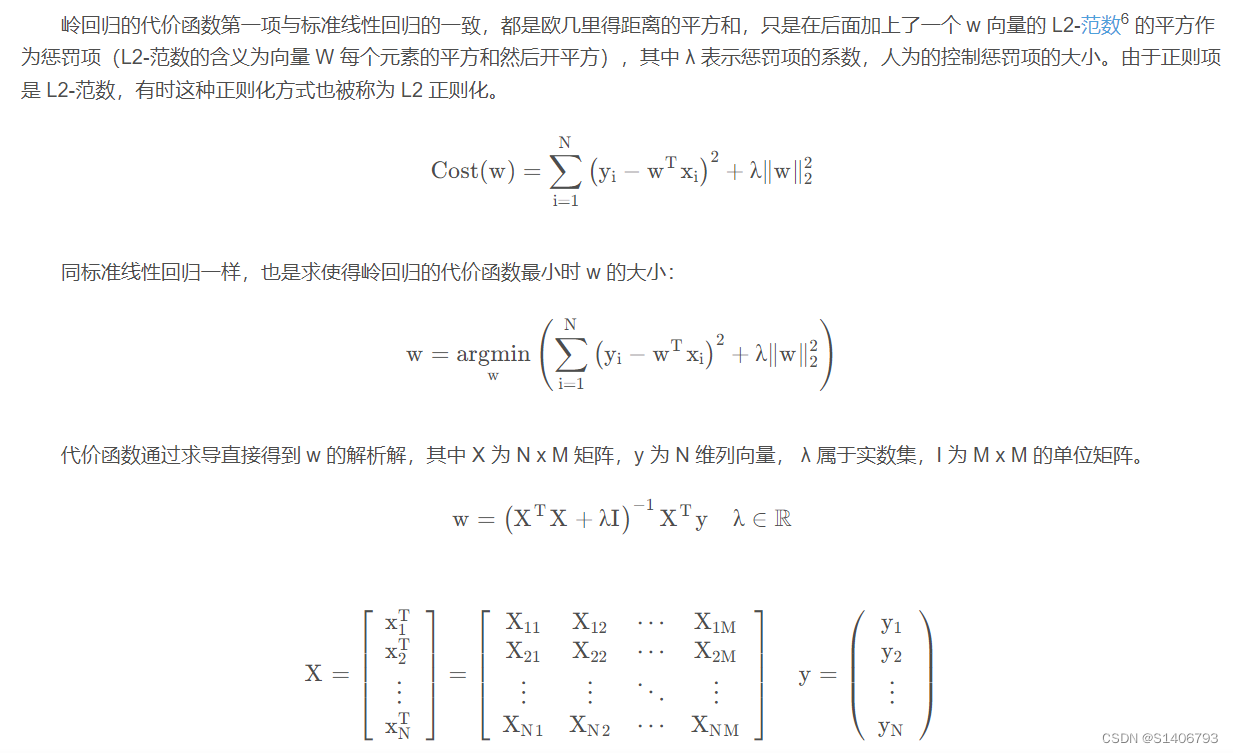• λ越大，系数值越小；λ越小，系数值越大。
• 在拟合数据时，使模型的权重尽可能小。
• 前面我们学习了标准线性回归算法，最后求得标准线性回归的代价函数的解析解，如果矩阵 X 的转置与矩阵 X 相乘后的矩阵不是满秩矩阵时，这个矩阵是不可逆的，其本质是多个自变量 x 之间存在多重共线性。
• 加了惩罚项后，相较于标准线性回归的结果，拟合可能会变差，但是通过人为的控制惩罚项的大小，可以解决自变量多重共线性的问题
• 多重共线性（Multicollinearity）是指多变量线性回归中，变量之间由于存在精确相关关系或高度相关关系而使回归估计不准确。

## 8.2 LASSO回归

我们学习了解决多重共线性的一种方法是对代价函数正则化，其中一种正则化的算法叫岭回归算法（Ridge Regression Algorithm）。下面我们来学习另一种正则化的算法 - Lasso回归算法1（Lasso Regression Algorithm）。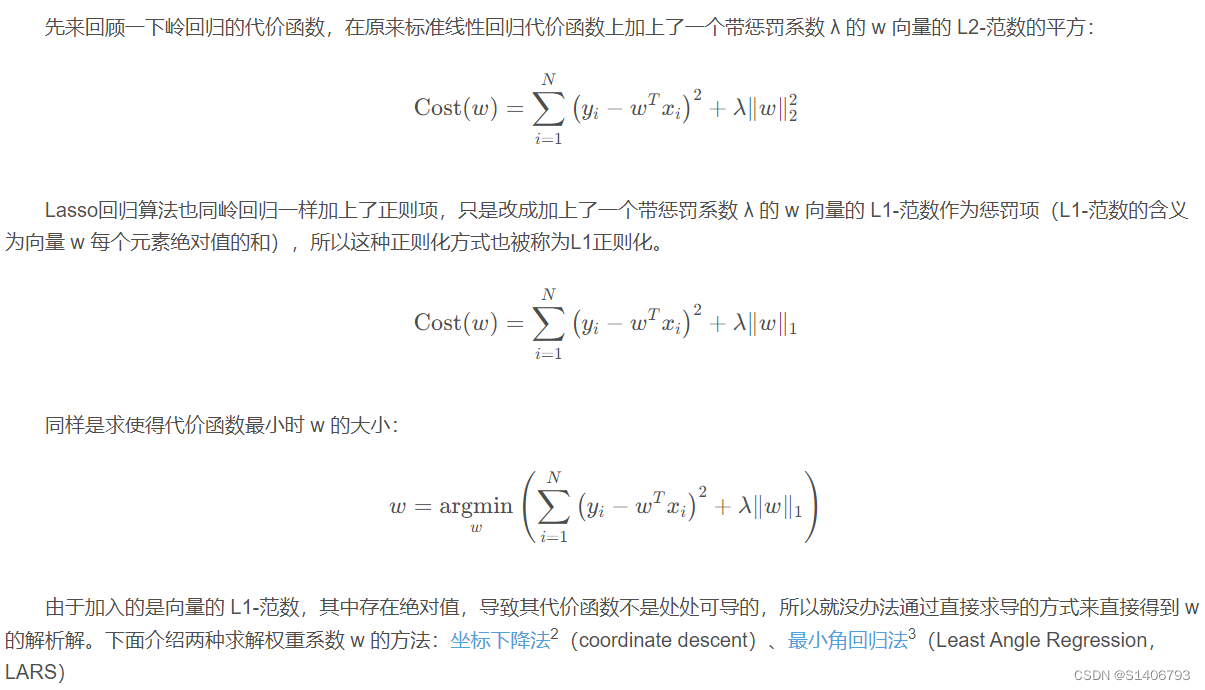• Lasso回归的代价函数在w=0处不可导（绝对值在顶点处不可导），所以进行计算的过程中产生很多0，很多特征的系数为0
• 解决方法：在w=0处用一个次梯度向量代替梯度。
• lasso回归的重要性质是：倾向于完全消除不重要的权重。
• 例如，当λ取值较大时，高阶多项式退化为二次甚至线性。 高阶多项式特征的权重置为0.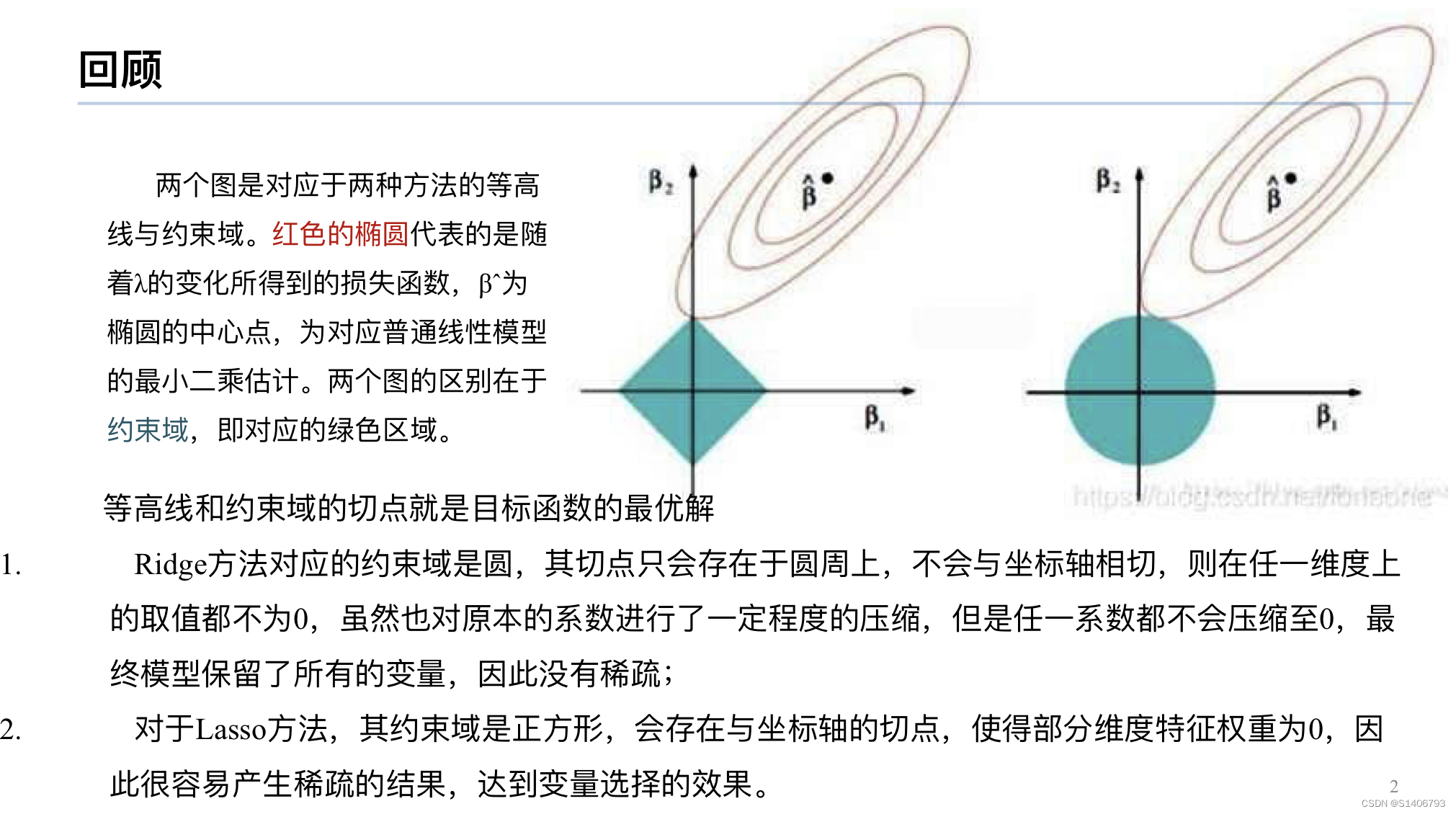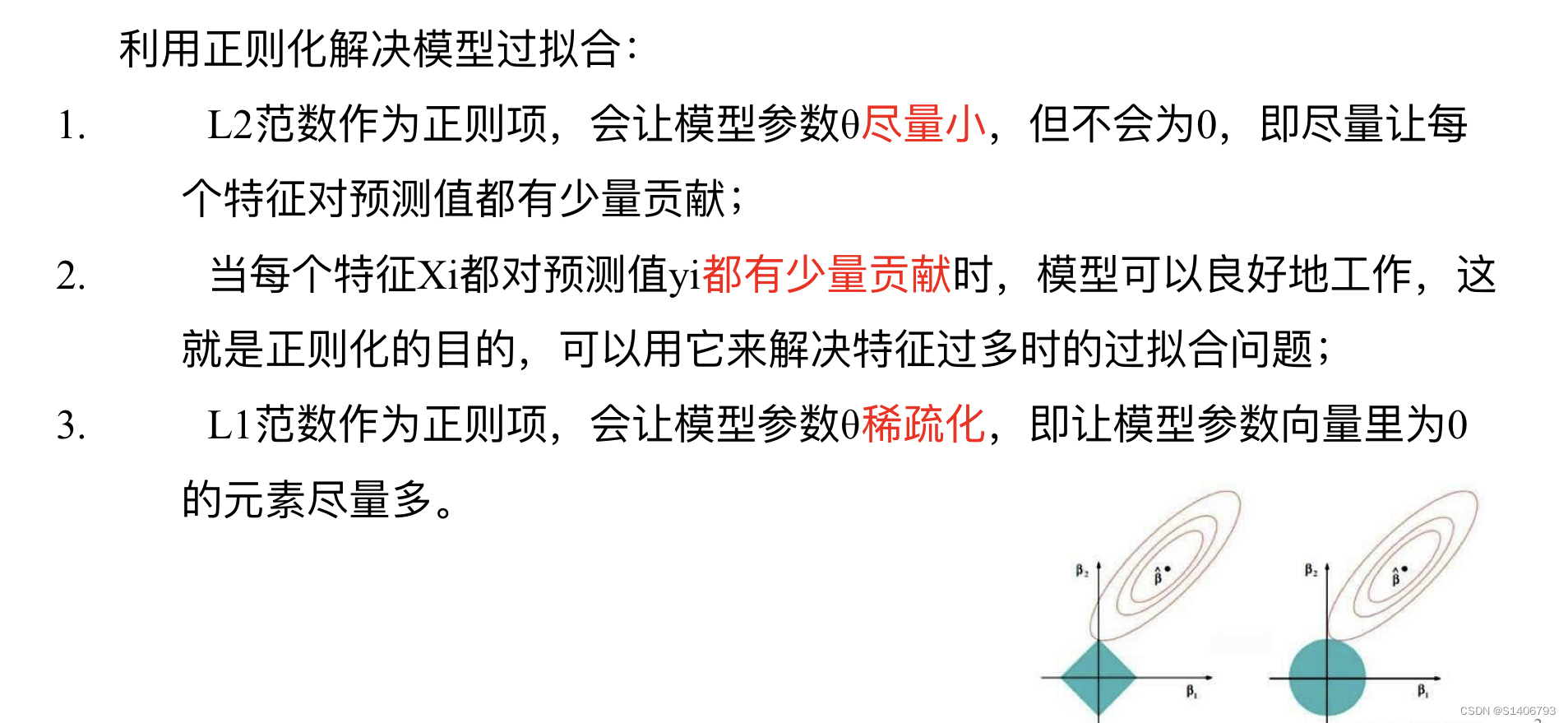## 8.3 Elastic Net弹性网络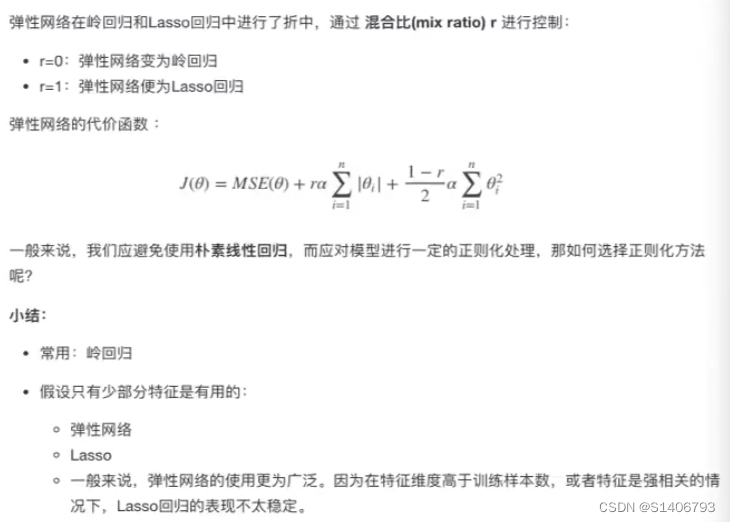API：

from sklearn.linear_model import Ridge, ElasticNet, Lasso

## 8.4 Early stopping [了解]

Early Stopping也是正则化迭代学习的方法之一

## 8.5 岭回归的应用——波士顿房价预测

• sklearn.linear_model.Ridge(alpha=1.0, fit_intercept=True, solver='auto', normalize=False)
• alpha:正则化力度，也叫λ，使用默认值即可
• λ取值：0-1  1-10
• solver：会根据数据自动选择优化算法，最多sag梯度下降
• sag：如果数据集、特征都比较大，选择该随机梯度下降法优化
• normalize：数据是否进行标准化
• normalize=True，就可以不用再另外fit调用preprocession.StandardScaler标准化数据
• Ridge.coef_：回归权重
• Ridge.intercept_：回归偏置

Ridge方法相当于随机梯度下降法（SGDRegression(penalty='l2', loss='squared_loss')），只不过SGDRegression实现了一个普通的随机梯度下降学习，推荐使用Ridge（实现了SAG：随机平均梯度下降法）

RidgeCV回归API：

• sklearn.linear_model.RidgeCV(_BaseRidgeCV, RegressorMixin)
• alpha对应的值可以通过网格搜索进行值的传递，默认alpha=（0.1,1.0,10.0），去尝试哪个值更好，这里的alpha就相当于lambda，正则化力度
• 具有L2正则化的线性回归，可以进行交叉验证
• coef_：回归系数
• normalize=True：默认封装了，对数据进行标准化处理，如果之前StandardScaler进行了标准化，就normalize=False
def _BaseRidgeCV(LinearModel):
def __init__(self, alphas=(0.1,1.0,10.0),    #alpha就是λ，正则化力度
fit_intercept=True, normallize=False, scoring=None,
cv=None, gcv_mode=None,
store_cv_values=False):

正则化力度越大，权重系数会越小； 正则化力度越小，权重系数会越大。

### 波士顿房价预测——Ridge、RidgeCV：

import pandas as pd
import numpy as np
from sklearn.model_selection import train_test_split  #数据基本处理
from sklearn.preprocessing import StandardScaler  #特征工程
from sklearn.linear_model import LinearRegression, SGDRegressor, Ridge #机器学习
from sklearn.externals import joblib #模型保存和加载
from sklearn.metrics import mean_squared_error   #模型评估

def linear_model3():
'''
线性回归：岭回归
'''
# 1.获取数据

# 2.数据基本处理
# 2.1数据集划分
x_train,x_test,y_train,y_test = train_test_split(boston.data, boston.target, test_size = 0.2)

# 3.特征工程: 实例化＋fit_transform方法
transfer = StandardScaler(x_train,x_test)
x_train = transfer.fit_transform(x_train)
x_test = transfer.fit_transform(x_test)

# 4.机器学习（线性回归）
# 4.1 模型训练
#estimator = Ridge(alpha=1)#岭回归
estimator = RidgeCV(alphas=(0.001,0.1,1,10.0,100.0)#RidgeCV，正则系数，自动选择

# 4.2 模型保存
joblib.dump(estimator,'./data/linghuigui.pkl')
estimator.fit(x_train,y_train)
print('这个模型的偏置是：\n',estimator.intercept_)

# 5.模型评估
# 5.1 预测值和准确率
y_pre = estimator.predict(x_test)
print('预测值是：\n',y_pre)

score = estimator.score(x_test,y_test)
print('准确率是:\n',score)

# 5.2均方误差
ret = mean_squared_error(y_test,y_pre)
print('均方误差是：\n',ret)

if __name__ == '__main__':
linear_model3()


通过比较正规方程、梯度下降法、岭回归(Ridge)三者的结果（均方误差大小），发现岭回归比梯度下降法好一些，但是没有正规方程好。但是只要比梯度下降法好就OK，因为岭回归使用了正则化且使用了SAG梯度下降法。所以以后选择随机梯度下降和SAG梯度下降时，优先选择SAG梯度下降。

# 九、模型的保存和加载

sklearn模型的保存和加载API

• from sklearn.externals import joblib
• 保存：joblib.dump(eatimator, 'file_name.pkl') #保存哪个训练模型eatimator，保存的名字(后缀是**.pkl)

实例在岭回归的波士顿房价预测案例中。将模型保存之后，注释掉模型训练和保存代码，利用

estimator = joblib.load('./data/test.pkl')#加载模型

### 怎样编写裸片启动程序-ARMv8的Boot Code和ROM程序

ROM程序就是固化在芯片的ROM里面&#xff0c;把应用程序从存储器里加载/搬移到RAM中并使处理器开始执行应用程序的一段程序 1 Boot Code和ROM程序 从多普通单核MCU&#xff08;如STM32&#xff09;的使用者的角度来看&#xff0c;只需要把编译好的hex文件烧写到片上Flash中&am…

### Redis 入门及应用 ( 五 ) 双写一致性

7.高级应用 7.1.数据库和缓存双写一致性 如果更新了数据库中的数据, 在缓存中的数据如何保持一致性? 4种方案 : 先写缓存, 再写数据库先写数据库, 再写缓存先删缓存, 再写数据库先写数据库, 再删缓存 7.1.1.先写缓存, 再写数据库 先将更新的数据写入缓存, 再写入到数据 …

### 【Pytorch Lighting】第 2 章：第一个深度学习模型起步

&#x1f50e;大家好&#xff0c;我是Sonhhxg_柒&#xff0c;希望你看完之后&#xff0c;能对你有所帮助&#xff0c;不足请指正&#xff01;共同学习交流&#x1f50e; &#x1f4dd;个人主页&#xff0d;Sonhhxg_柒的博客_CSDN博客 &#x1f4c3; &#x1f381;欢迎各位→点赞…

### 【生日快乐】Node.js 实战 第1章 欢迎进入Node.js 的世界 1.2 ES2015、Node 和 V8

Node.js 实战 文章目录Node.js 实战第1章 欢迎进入Node.js 的世界1.2 ES2015、Node 和 V81.2.1 Node 和 V81.2.2 使用特性组1.2.3 了解Node 的发布计划第1章 欢迎进入Node.js 的世界 1.2 ES2015、Node 和 V8 对于“以前的JavaScript” &#xff0c;Node解决了很多问题&#x…

### Excel - 字符串处理函数：LEFT, RIGHT, MID, LEN 和 FIND

Excel字符串处理函数&#xff1a;LEFT, RIGHT, MID, LEN 和 FIND。 我们在Excel里会存储一些字符串&#xff0c;其内容一般包含&#xff1a;字符、数字、符号(比如横线符 "-")&#xff0c;以及空格等。 如果我们需要从中要获取一部分内容&#xff0c;那如何在Excel中…

### 70.qt quick-QQmlListProperty详解

qml与c++少不了类表传递、比如我们之前的好友列表、文件传输列表等等、用的是QListModel、QList类指针表传递、QVariantList等、本章我们使用一个新的传递方式QQmlListProperty、 本章源码已上传至专栏群(第一章底部获取)中,感兴趣的自行下载、 1. QQmlListProperty介绍 QQml…

### 【生日快乐】SpringBoot SpringBoot 提高篇(第二篇) 第6章 SpringBoot 高级配置 6.1 临时属性配置

SpringBoot 【千锋教育java教程SpringBoot2全套&#xff0c;springboot快速入门到项目实战视频教程】 SpringBoot 提高篇(第二篇) 第6章 SpringBoot 高级配置 文章目录SpringBootSpringBoot 提高篇(第二篇)第6章 SpringBoot 高级配置6.1 临时属性配置6.1 临时属性配置 创建…

### 比Linus更牛逼的程序员，QEMU、FFmpeg的作者

Linus Torvalds是个非常厉害的程序员&#xff0c;因为他有两个名扬天下的作品&#xff1a;Linux和Git。 如果单论技术能力&#xff0c;有一个人&#xff0c;也许比Linus更强。 我在看他主页项目列表的时候&#xff0c;感觉头都炸了。 他开发了著名的模拟器QEMU和音视频处理库…